# Maths - Internal Language of a Topos

#### Language and Interpretation

The Mitchell-Bénabou language has a syntax and this can be translated into an interpretation.

The interpretation is a function. When we are dealing with logic then these are functions into the truth object Ω but they can be mixed in with other types of function.

This function into the truth object Ω is like a predicate. An example of this might be an equation like: x=3 or a predicate like even(x). These both give a value which is true or false for any given value of x. Here we are generalising from set theory/Boolean values to Topos theory/truth objects. So we have something that looks like a mapping from a set of variables to the truth object.

## Mitchell-Bénabou Language

see ncatlab

A simple form of the internal language of an elementary topos E. It makes use of the fact that in the presence of a subobject classifier Ω, there is no need to treat formulas separately from terms, since a formula or proposition can be identified with a term of type Ω.

#### Table

Term Interpretation (arrow)
T1

Variables

May be free or bound as in lambda calculus.

I1

Variable xi in list
x
=(x1,x2,x3...xn)

For a free variable xi the interpretation is the ith projection.T2

Arrow

f: x->y

I2

The interpretation of f s is f |s|.

|f s| -> f |s|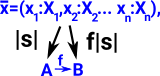T3

Constant

c: 1->a

I3 The interpretation of a constant 'c' is c!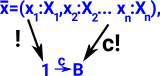T4

Product

For a term:

• s1 of type A
• s2 of type B

there is a term <s1,s2> of type A×B.

I4

The interpretation of a product <s1,s2> is <|s1|,|s2|>

|<s1,s2>| -> <|s1|,|s2|>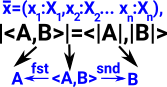T5

Exponential

For a term s of type B and a term y of type Y there is a term λ( y.Y)s of type BY.

We say the variable y is bound by the lambda operator.

I5

|λ( y.Y)s |x,y-> <|s|x>

where s is transpose of s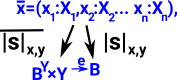### Transpose

The transpose above in exponential may need more explanation. See Currying.

We can replace

f(x,y) -> R

with

f(x) -> (y -> R)

which can be denoted:

f(x) -> RY

So input parameters can be removed provided the output format is changed.

### Internalising Structure

In type theory an element of a type is made (instantiated) using a type constructor. Can we customise these types (build structure into the type) by having constraints in addition to the constructors?

Denoted as an extension like this:

[constructors | constraints]

An extension is the subobject classified by the map into Ω (constraints).

Similar idea to group presentation.

where each contraint is a function from the variables to the truth object (as above).

## Subobject Classifier

 A subobject classifier is an arrow from 1 (terminal object) to Ω.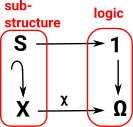This is an extension. It can be thought of like a comprehension where the variables are before the '|' and the classifier (which specifies a sub-object) is after it.

 [x.X | χq(x) /\ χr(x) ]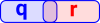[x.X,y.Y | χq(x) /\ χr(y) ]### Model Theory

All the flavours of model theory rest on one fundamental notion, and that is the notion of a formula φ being true under an interpretation I. (Wilfrid Hodges)

#### Theory

In model theory a 'theory' is a set of first-order sentences, for instance:

•xyz.(x+(y+z)=(x+y)+z)
•x.(x+0=x)
•x.(x+(−x)=0)
•xy.(x+y=y+x)

#### Model of Theory

There can be multiple models of any given theory

### Internal Logic

See ncatlab:

large parts of mathematics can be internalized in any category with sufficient structure

The idea is to exploit the fact that all mathematics can be written in the language of logic, and seek a way to internalize logic in a category with sufficient structure.

The basic ideas of the internal logic induced by a given category C are:

 The objects A of C are regarded as collections of things of a given type A: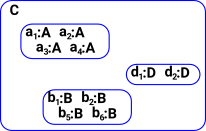The morphisms A->B of C are regarded as terms of type B containing a free variable of type A (i.e. in a context x:A)A subobject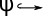A is regarded as a proposition (predicate): by thinking of it as the sub-collection of all those things of type A for which the statement φ is true the maximal subobject is hence the proposition that is always true; this is the logical object of truth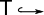A the minimal subobject is hence the proposition that is always false; this is the logical object of falsity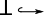A one proposition implies another if as subobjects of A they are connected by a morphism in the poset of subobjects: φ=>ψ meansψ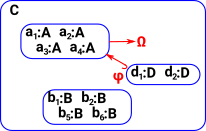Logical operations are implemented by universal constructions on subobjects. the conjunction and is the product of subobjects (their meet) the conjunction or is the coproduct of subobjects (their join) A dependent type over an object A of C may be interpreted as a morphism B->A whose “fibers” represent the types B(x)for x:A. This morphism might be restricted to be a display map or a fibration.

### Alternative Version of Expression

variables -> truth value

x1,x2,x3...xn -> Ω

## Substitution as Pullback

Can we express substitution in category theory terms? See discussion on page here.

 Substitution of a term into a predicate is pullback, but substitution of a term into a term is composition. Type theory version Substitution of a term into a dependant type is pullback, but substitution of a term into a term is composition.

There is a discussion of this topic on this site.

So why the difference? I think in the predicate case we are going backwards.

 Typically a predicate could be an equation which may be true or false depending on the values of its variables. Or a function from a variable to bool (or some truth object).